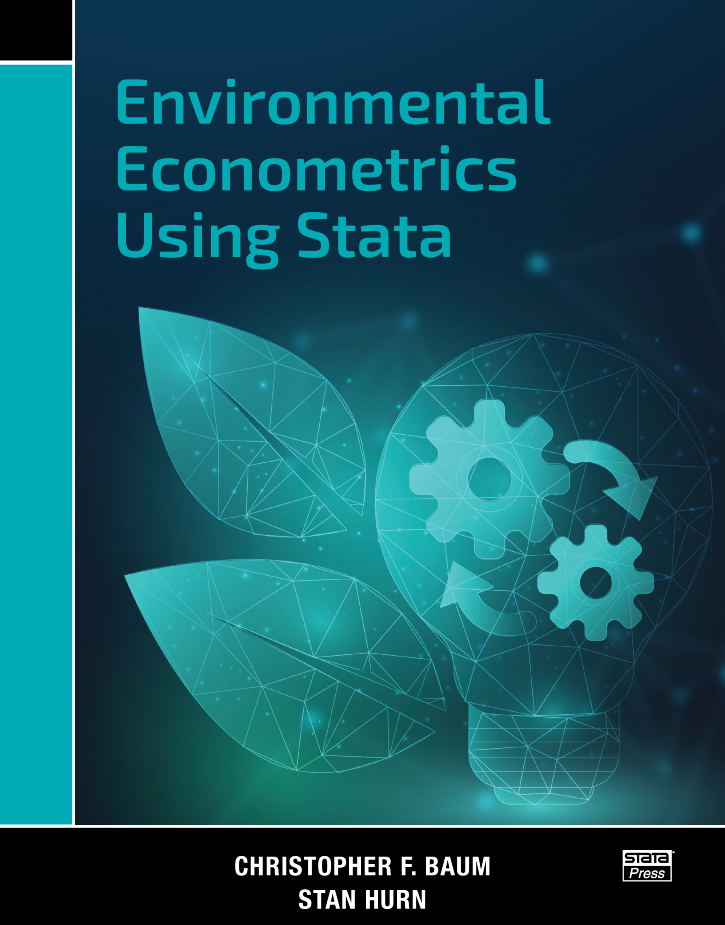# Environmental Econometrics Using Stata

Environmental Econometrics Using Stata is written for applied researchers that want to understand the basic theory of modern statistical methods and how to use them. It is also perfectly suited for teaching. Each chapter is motivated with real data and ends with a set of exercises. The book is also inherently interdisciplinary. The questions posed by environmental issues are relevant to researchers in the physical sciences, economics, sociology, political science, and public health, among other fields.

Each chapter begins with a real dataset and research question. The authors then provide a gentle introduction to the statistical method and demonstrate how to use it to answer the research question. The authors discuss the assumptions about the data and the model, demonstrate the Stata commands used to fit the model and check the model assumptions, and interpret the results. The workflow of the book mimics the workflow that would be required to present your results to an academic audience.

The book is of interest not only for its exposition of the topics but also for its breadth. The book presents estimators for continuous, binary, and ordered outcomes in cross-sectional data; univariate and multivariate time series with stationary and nonstationary data; linear and dynamic panel data; and spatial models and fractional integration. The range of methods is not arbitrary; it is a function of the questions posed by environmental data and reflects the challenges faced by researchers from different disciplines to answer a wide range of questions using modern statistical methods.

List of figures
List of tables
Preface
Acknowledgments
Notation and typography

1. INTRODUCTION
Features of the data
Periodicity
Nonlinearity
Structural breaks and nonstationarity
Time-carying volatility
Types of data

2. LINEAR REGRESSION MODELS

Air pollution in Santiago, Chile
Linear regression and OLS estimation
Interpreting and assessing the regression model

Goodness of fit
Tests of significance
Residual diagnostics
Homoskedasticity
Serial independnece
Normality

Estimating standard errors

3. BEYOND ORDINARY LEAST SQUARES

Distribution of particulate matter
Properties of estimators
Consistency
Asymptotic normality
Asymptotic efficiency

Maximum likelihood and the linear model
Hypothesis testing

Likelihood-ratio test
Wald test
LM test

Method-of-moments estimators and the linear model
Testing for exogeneity

4. INTRODUCING DYNAMICS

Specifying and fitting dynamic time-series models
AR models
Moving-average models
ARMA models
Exploring the properties of dynamic models
ARMA models for load-weighted electricity price
Seasonal ARMA models

5. MULTIVARIATE TIME-SERIES MODELS

CO2 emissions and growth
The VARMA model
The VAR model
Analyzing the dynamics of a VAR
Granger causality testing
Impulse-responses
Vector moving-average form
Orthogonalized impulses
Forecast-error variance decomposition
SVARs
Short-run restrictions
Long-run restrictions

6. TESTING FOR NONSTATIONARITY
Per capita CO2 emissions
Unit roots
First-generation unit-root tests
Dickey—Fuller tests
Phillips—Perron tests
Second-generation unit-root tests
KPSS test
Elliott—Rothenberg—Stock DFGLS test
Structural breaks
Known breakpoint
Single-break unit-root tests
Double-break unit-roots tests

7.  MODELLING NONSTATIONARY VARIABLES
Illustrating equilibrium relationships
The VECM
Fitting VECMs
Single-equation methods
System estimation

Testing for cointegration
Cointegration and structural breaks

8. FORECASTING
Forecasting wind speed
Introductory terminology
Recursive forecasting in time-series models
Single-equation forecasts
Multiple-equation forecasts
Properties of recursive forecasts

Forecast evaluation
Daily forecasts of wind speed for Santiago
Forecasting with logarithmic dependent variables

Staying in the linear regression framework
Generalized linear models

9 STRUCTURAL TIME-SERIES MODELS
Sea level and global temperature
The Kalman filter
Vector autoregressive moving-average models in state-space form
Unobserved component time-series models
Trends
Seasonal
Cycles
A bivariate model of sea level and global temperature

10. NONLINEAR TIME-SERIES MODELS
Sunspot data
Testing
Bilinear time-series models
Threshold autoregressive models
Smooth transition models
Markov switching models

11. MODELLING TIME VARYING VARIANCE
Evaluating environmental risk
The generalized autoregressive conditional heteroskedasticity model
Alternative distributional assumptions
Asymmetries
Motivating multivariate volatility models
Multivariate volatility models
The vech model
The dynamic conditional correlation model

12. LONGITUDINAL DATA MODELS
The pollution haven hypothesis
Data organization
Wide and long forms of panel data
Reshaping the data
The pooled model
Fixed effects and random effects
Individual FEs
Two-way FE
REs
The Hausman test in a panel context
Correlated RE
Dynamic panel-data models

13. SPATIAL MODELS
Regulatory compliance
The spatial weighting matrix
Specifications
Distance weights
Contiguity weights
Construction
Exploratory data analysis
Spatial models
Spatial lag model
Spatial error model

Fitting spatial models by maximum likelihood

Spatial lag model
Spatial error model

Estimating spillover effects
Model selection

14. DISCRETE DEPENDENT VARIABLES
Humpback whales
The data
Binary dependent variables
Linear probability model
Binomial logit and probit models

Ordered dependent variables
Censored dependent variables

15. FRACTIONAL INTEGRATION
Mean sea levels and global temperature
Autocorrelations and long memory
Testing for long memory
Estimating d in the frequency domain
Maximum likelihood estimation of the ARFIMA model
Fractional cointegration

A USING STATA
File management
Organization of do-, ado-, and data files
Basic data management
Data types
Handling text files
The import delimited command
Importing data from other package formats
Other data issues
Protecting the data in memory
Missing data handling
Recoding missing values: the mvdecode and mvencode commands
String-to-numeric conversion and vice versa
General programming hints
Variable names
Observation numbering: n and N
The varlist
The numlist
The if exp and in range qualifiers
Local macros
Global macros
Scalars
Matrices
Looping
The generate command
The egen command
Computation for by-groups
A smorgasbord of important topics
Date and time handling
Time-series operators
Factor variables and operators
Circular variablesAuthor: Christopher F. Baum and Stan Hurn
ISBN13: 978-1-59718-355-0